August 11, 2022
Bert Christiaens

## Using generative AI for image manipulation: discrete absorbing diffusion models explained

Computer Vision

Within machine learning, there is one class of computer vision models that can give results with an unparalleled ability to astonish: the generative models. These models can change the style of an image, imagine missing parts and much more. They show us how much deep learning has improved in learning from data but also generating it.

Generative models for computer vision usually work by sampling vectors from a learned distribution, the latent space, and project them into image space with a decoder model. Although this results in high quality images, these models generally give a limited control over the latent space, making it hard to guide the generation process.

Various methods have risen to solve this problem: conditioning on segmentation maps (GauGAN), text prompts (DALL-E), style vectors (StyleGAN), … and allow the user to specify some details about the wanted result. Unfortunately, these still don’t give fine-grained control over the latent space. In this blogpost, we will discuss a new technique called Discrete Absorbing Diffusion models, which gives us various options to steer the generative model and improve the usability for creative applications.

In this blogpost we’ll start with some recent advances in Deep Learning, such as Transformers (vaswani et al. 2017) and VQGANs, and finally take a deeper look at the Discrete Absorbing Diffusion model from: Unleashing Transformers: Parallel Token Prediction with Discrete Absorbing Diffusion for Fast High-Resolution Image Generation from Vector-Quantized Codes.

If you want to see the creative applications that this architecture allows, you can read the Applications blogpost!

## Understanding VQVAE

There exist several models that can generate new data. The most widely known families of models are GANs, flow models, autoregressive models and variational autoencoders. Today we will take a quick high-level look at the family of autoencoders, and then take a deep dive into the VQVAE.

### Vanilla autoencoder (AE)

The family of autoencoders starts with a non-generative model, the vanilla autoencoder. The idea behind this model is to compress the input data, such as images, into a conceptual (usually lower dimensional) representation that contains most of the information of the input. Its objective is to reconstruct the original input from this compressed intermediate (or latent) representation, by feeding it through a decoder network.

Although this encoder-decoder architecture was innovative, it has the limitation that you could not sample values from this latent space and generate new images. This is because we don’t know which values of the latent space correspond to sensible outputs, so many combinations of latent values will result in nonsense.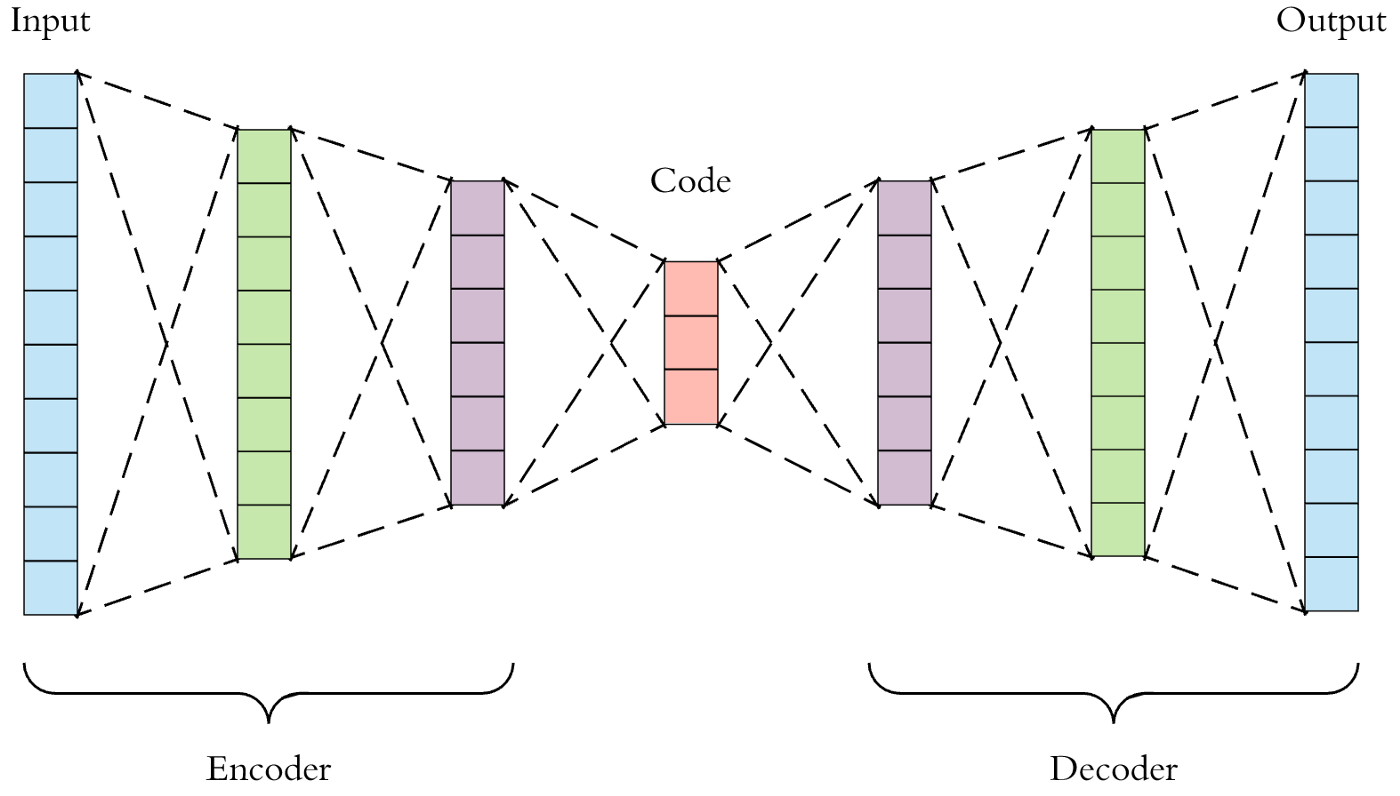The vanilla autoencoder: the input is projected onto a a low-dimensional latent space (the code) by the encoder. Then the decoder projects this code back into image space in order to reconstruct the input values. https://towardsdatascience.com/applied-deep-learning-part-3-autoencoders-1c083af4d798

### Variational Autoencoder (VAE)

To solve this problem, we have to enforce structure in the latent space. The VAE achieves this by adding the objective that the latent variables outputted by the encoder must approximate a prior distribution. This is realised by including the Kullback–Leibler divergence in the loss function, which measures how much a distribution differs from another distribution. By regularising this latent space, the latent variables become more smooth and meaningful.

Now we know that a sample from this prior distribution (if trained well) results in an image similar to the training data. Finally we have a real generative model!

### Vector Quantized VAE

A newer member of the auto-encoder family is the Vector Quantized VAE (VQVAE), which takes a slightly different approach, namely it represents the latent space by using a grid of discrete codes.

So how do we go from continuous latent variables to discrete codes?

The VQVAE achieves this by using a new component: the codebook. The idea is that you learn a fixed amount of vectors that are allowed in the latent space. First, the encoder processes the input and outputs a grid of vectors. These vectors are then compared to the codes in the codebook and are replaced with the closest code (by using L2 distance), which is called the vector quantization step.

Each vector of the grid now corresponds to one of the codebook vectors and can be assigned an index corresponding the location in the codebook. This results in a discretization of the latent space, opposed to the continuous nature of the VAE. As with all autoencoders, the decoder takes the latent variables and maps them back to an image.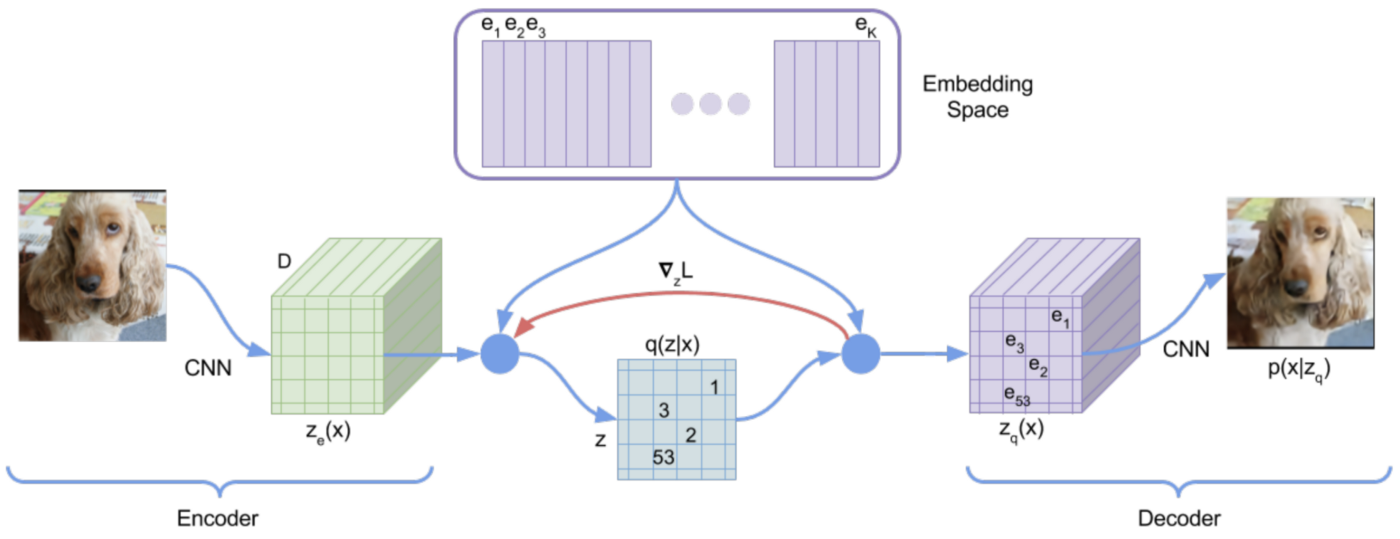Architecture of the VQVAE: image is encoded into a grid of latent vectors. These vectors are replaced by the closest vector in the codebook in the bottleneck. Finally, the quantized vectors go through the decoder to reconstruct the input.

### Why do we care about discrete spaces?

Very cool, but why go through all the trouble to constrain the latent space?

• Going from vectors with floating point values to discrete indices further compresses the latent space, giving us a compact representation.
• By using maximum likelihood instead of a variational objective, the training becomes more stable.
• By using the whole latent space in the bottleneck, we avoid posterior collapse. This is a common problem in VAEs, where the decoder ignores a subset of the latent variables.
• By discretizing the latent space with the codebook, we get a natural representation, somewhat like a language of visual concepts. The discrete representations also allow the use of very powerful models such as the famous transformers.

This is a high-level explanation that omitted some information concerning the architecture and training of VQVAEs. If you would like to learn more, take a look at one of the following explanations:

## VQVAE metaphor: the architect

So let us try to find a good metaphor for the encoder, codebook, latent space and decoder.

You want to build your own church and go to an architect that knows everything about churches. In your head you approximately know how you want your building but you are only able to describe it. The architect tells you: here I have a catalogue of every element that can occur in a church (aka the codebook), show me where I should put which one. You convert (aka you encode) the image in your head into this small grid by using the catalogue elements (a roof goes here, the door goes there, …).

Since the architect (aka the decoder) is so experienced and knows how these elements should form a building, he can now decode your high-level schema (aka the latent space) into a beautiful representation of the church. And wow, it looks just like you imaged!

This is the main idea of the VQVAE: the latent space represents a conceptual spatial overview using elements (discrete codes) from the codebook. The decoder is then able to project this low-dimensional (for example 16x16) overview into a high-dimensional (256x256) image.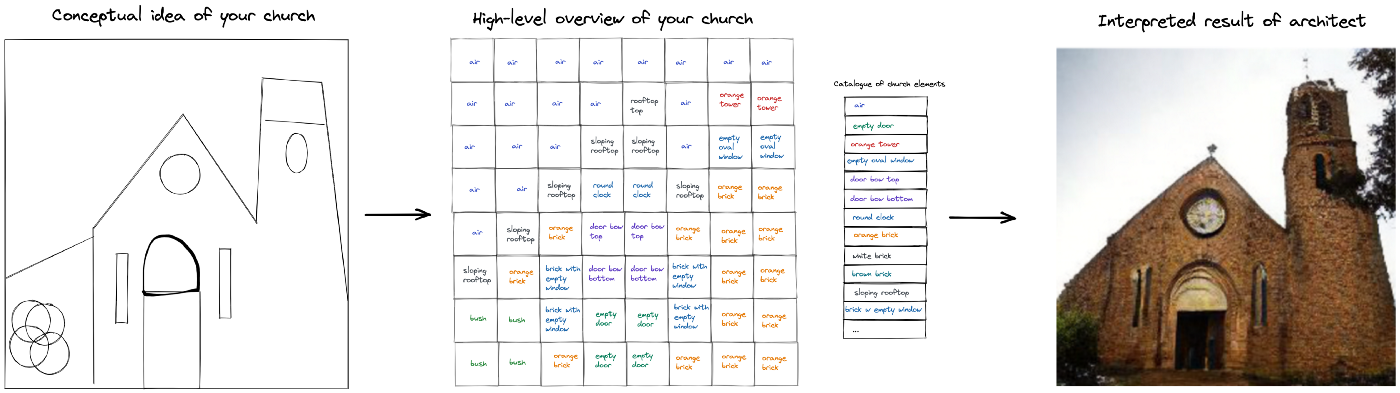Metaphor of the VQVAE latent space and codebook. The codes represent a catalogue of high-level concepts, learned from the data, that can be used to construct a conceptual representation of an image. The decoder (the architect) knows how to interpret and map the small conceptual grid back to image space.

## Improving VQVAE with an adversarial framework

One thing a VAE is good at is obtaining high likelihood (meaning the reconstruction are good on average). However this can mean that the VAE tries to minimize the errors on average by playing it safe and predicting an on average ‘safe’ pixel value, resulting in blurry images.

To avoid these blurry images, we can get some inspiration from Generative Adversarial Networks (GANs), where the objective is to make realistic samples that can fool a discriminator model. The VQGAN adds a discriminator on top of the VQVAE that predicts whether each image patch is real or generated. This added adversarial loss encourages the VQVAE decoder to produce sharp and realistic samples. For a more in-depth explanation, take a look at this paper.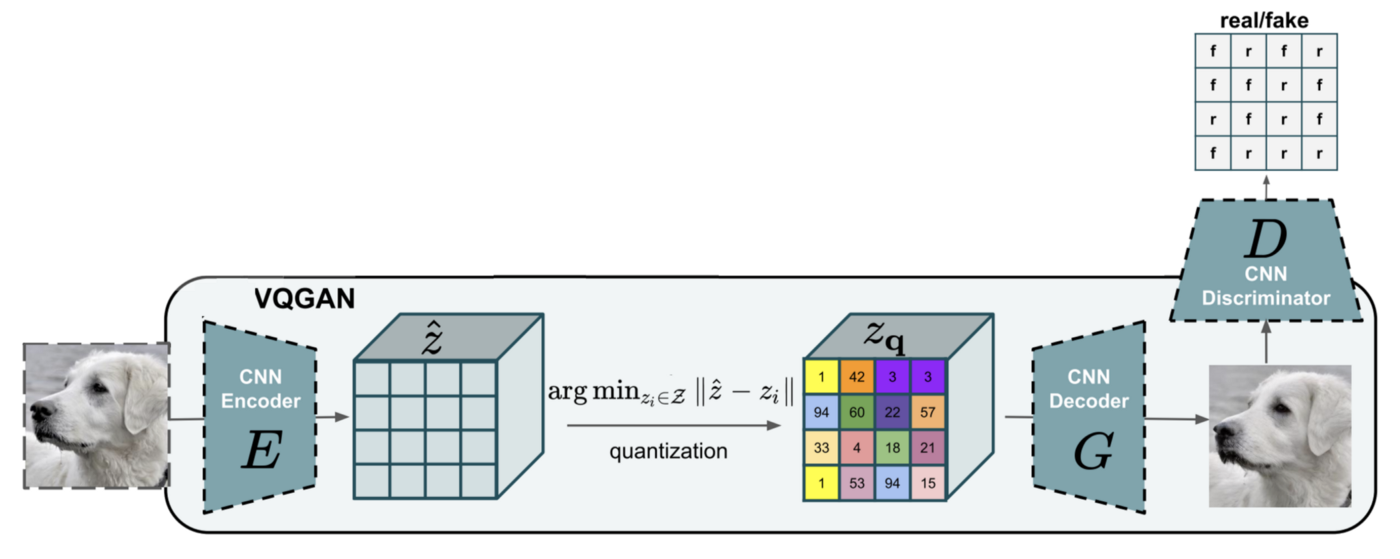Architecture of the VQGAN: a discriminator model looks at images generated by the VQVAE to predict whether each patch is real of fake.

## Sampling from VQVAE

We have discussed what a VQVAE is and how it uses a discrete representation to represent images. However, to generate data, we need some way to generate new combinations of latent codes that produce sensible images when decoded.

### Prior distribution

A first idea is to use a prior distribution to sample from the latent variables, similar to the Variational Autoencoder.

Since we are dealing with discrete variables, we can use a uniform prior on the discrete codes, so that each code has the same probability of being chosen in the grid. However, in reality this results in very inconsistent and low quality samples.

Looking at our metaphor, you can’t just randomly place a window in the air and a roof under your door and expect the architect to create something logical. This comes from the fact that in the original data distribution, the codes are not independent and the ‘appropriateness’ of a code depends on which codes are already present. We need a way of better representing the distribution of discrete codes in order to create coherent images.

### Learning the prior

To address this sampling problem, the original VQVAE paper proposed to learn the prior distribution instead of using a fixed uniform distribution.

However, instead of learning the joint distribution directly, which is infeasible due to the enormous amount of combinations that have to be learned, we would like to split the distribution into multiple smaller conditional probabilities.

### Autoregressive models

Autoregressive (AR) models solve this problem by taking advantage of the chain rule of probability:

Applied to our discrete latent space, we have to roll out the NxN grid of variables into a 1-dimensional array of length N². At each step, we only need to model the probabilities of the next variable by looking at the previous variables (conditional probability).

To model these factorized probabilities, the VQVAE paper used the autoregressive pixelCNN model. This model uses a masked sliding window, that looks at surrounding variables, to predict the next variable. Compared to our simple uniform prior, this approach improves the ability to generate new consistent images drastically by learning which codes occur together.

In the paper Taming transformers for high resolution image synthesis, the modelling of the prior is further improved by using a transformer decoder as autoregressive model.

A transformer decoder (such as GPT-2, GPT-3, …) takes in a sequence of tokens and predicts the next token in the sequence. This architecture can model long-range dependencies in a sequence, since its attention mechanism allows it to look at each token in the sequence. This is a big improvement over the pixelCNN, that can only look at a part of the information, due to its sliding window.

This transformer thus allows a better modelling of the relations between the latent codes while leveraging the compact representation and image reconstruction capabilities of the VQGAN.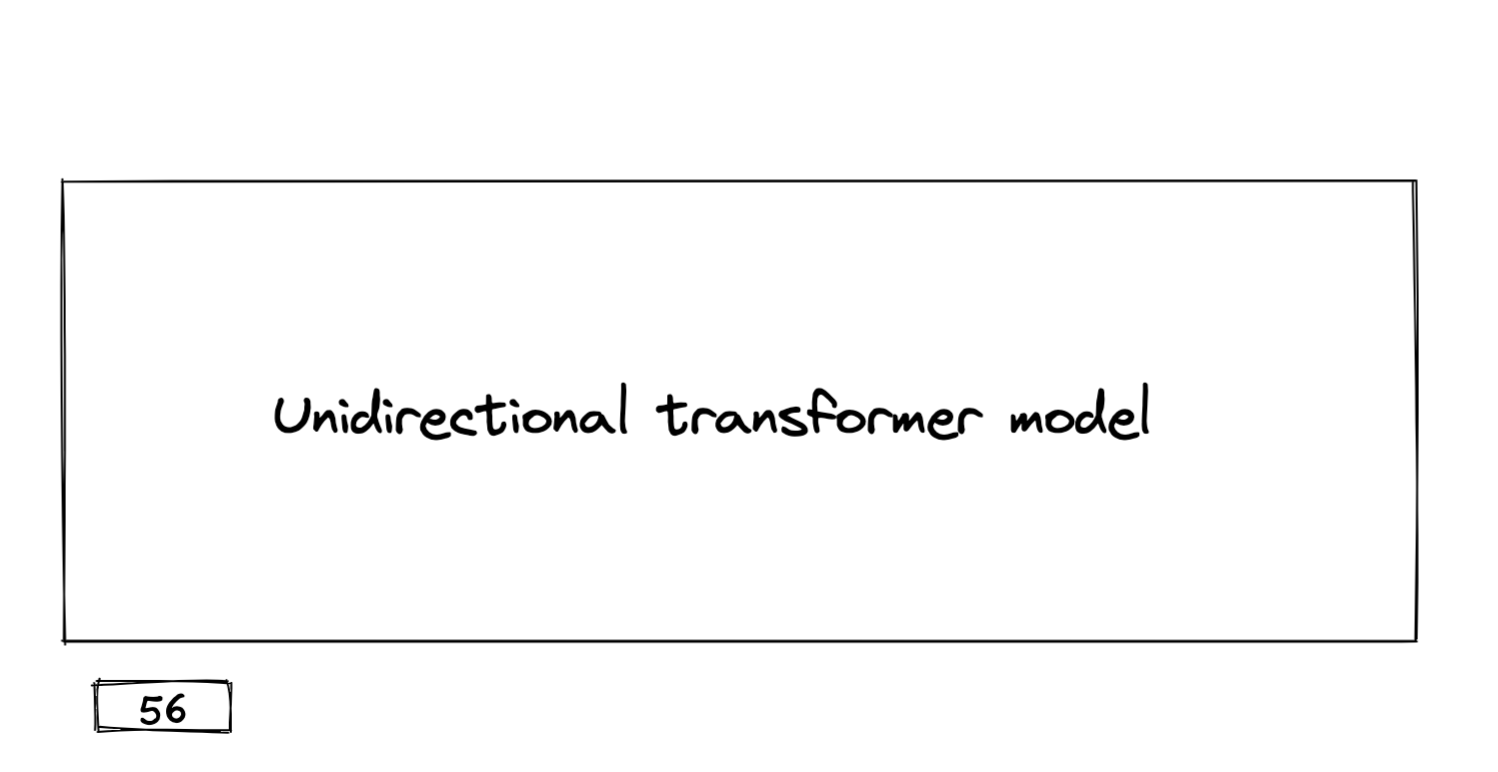Autoregressive generation of discrete codes with the transformer decoder model

#### Limitations

Although this architecture is pretty awesome and combines multiple SOTA techniques, it still has its limitations: it uses a constrained unidirectional transformer model that generates codes in a left-to-right manner. However, images are not naturally structured this way, which could lead to a lack of global consistency of the generated images. Since the codes are generated one-by-one, this sequential process is much slower than GANs and VAEs, that produce samples in a single pass through the network.

## Diffusion models

So how can we solve the issues of global consistency and sample speed?

• To improve the global consistency, the generation of new tokens should not be unidirectional but bidirectional. We want to look at the full context and the model should be allowed to produce a new token at some chosen location. This way, the global structure of the image can be chosen first and the rest can be filled in afterwards to complete the image.
• To improve the sample speed, we should get rid of generating 1 token per step and be allowed to generate multiple ones if wanted.

These two solutions are proposed in the paper ‘Unleashing transformers’ which researches Discrete Absorbing Diffusion models.

### Diffusion models

The main idea for diffusion models is to gradually corrupt the data and to learn a model that can inverse this corruption process.

The hypothesis is that if you remove a very small part of information from data, you could predict which information was removed and restore the original data.

We will make this more concrete (and a bit more technical) and look how a continuous diffusion process is applied to images. It consists of a forward diffusion process and a denoising process.

#### Forward diffusion process

As discussed above, we first need to corrupt the information in the data. This is done by the forward diffusion process, which defines a markov chain

that adds small amounts of, typically scaled gaussian, noise to the image over a large amount of steps. This process iteratively destroys the information in the original image, which leaves us with nothing but gaussian noise at the end.Noise is added to the original image (left) at each step until the sample is pure noise.

#### Denoising process

Alright, we have made our image unrecognizable, but why don’t we do this in one big step? Since our image only loses small amounts of information each step, it’s not unthinkable that a model can learn to remove this noise. In other words, a model can be trained to reverse the noising process and predict the data at step T-1 from step T.

To make new unseen samples from this model, we just need to sample random noise and denoise it with by leveraging our reversed markov chain. If trained well, we will end up with a plausible image from our data distribution after N denoising steps.

### Discrete absorbing diffusion

So how do we make a discrete variant of the noising process? By absorbing information of course! Discrete absorbing diffusion does not add random continuous noise but selects a subset of the discrete variables (in our case, the VQGAN latent codes) to mask out at each step. This again gradually destroys the information in the data, since we are left with a fully masked image after enough steps.

As before, a model can then be optimized to model the reverse process, meaning it has to predict which discrete variable originally was behind each mask.

The paper choses a bidirectional encoder transformer model, similar to NLPs beloved BERT, that takes in all the tokens. The benefit of using a bidirectional model is that it can look at all the tokens (of which a subset is masked) and use this global information to unmask tokens. At each step, we choose a random masked token and predict which code it originally had. After N² steps (the size of the latent grid), each token is predicted and the produced grid of codes can be decoded by the VQVAE decoder to produce an image.

### Speeding up inference

To speed up the process you can unmask multiple (K) tokens in one step, which reduces the amount of sampling steps by a factor of K. This technique gives the user control over the quality-speed trade-off.

In the table below we see this trade-off by comparing the FID for different amounts of denoising steps (50, 100, 150, 200 and 256). For the Churches dataset, going from 256 to 150 steps only adds 0.23 to the FID metric, while being almost double as fast. Going to 50 steps increases this metric by 1.28 and is 5 times faster.